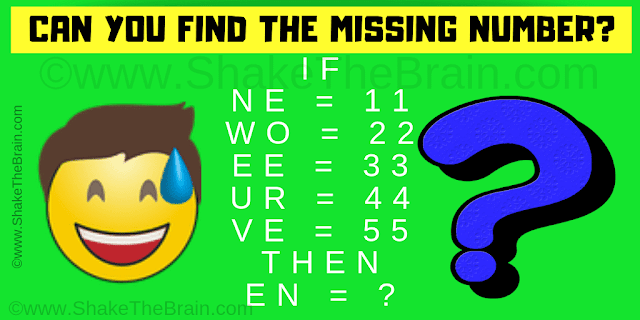This is a very simple and interesting maths logic problem which will shake your brain. Solving this maths logic puzzle will help you to improve your mathematical and logical reasoning skills. In this maths logic puzzle, your challenge is to study the given logical equations and then find the value of the missing number which will replace the question mark. Can you find the value of the missing number?Can you find the missing number?
The answer to this "Maths Logic Problem", can be viewed by clicking on the answer button.